Get instant live expert help with Excel or Google Sheets“My Excelchat expert helped me in less than 20 minutes, saving me what would have been 5 hours of work!”

#### Post your problem and you'll get expert help in seconds

Your message must be at least 40 characters
Our professional experts are available now. Your privacy is guaranteed.

# Split text string at specific character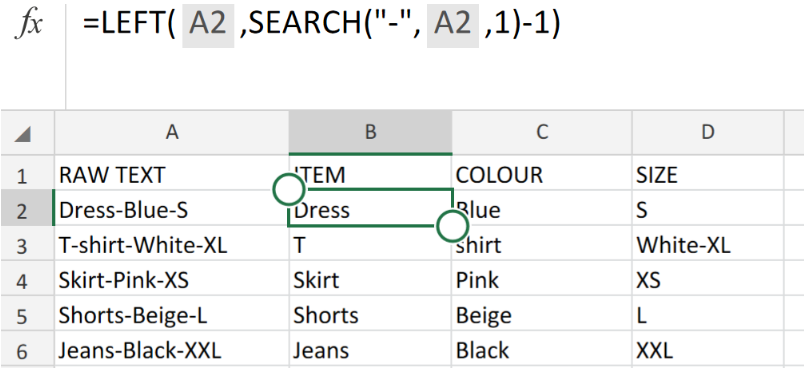Figure 1. of Split Text Function in Excel.

By making use of the Excel Right, Mid, Left, LEN and SEARCH Functions, we are able to manipulate of data in form of text.

This post will help us illustrate how to use different combinations of the Right, Mid, SEARCH and LEN Functions to split a string of text at a specific character.

## How to Split Text String by using Functions in Excel.

In the example illustrated below, we will be working with raw text string obtained from a clothing store. The storekeeper would like to have the data sorted and separated into different Excel sheet columns.

This can be done in 2 simple steps.

1. Set up your Excel sheet and input the requirements for the data splitting.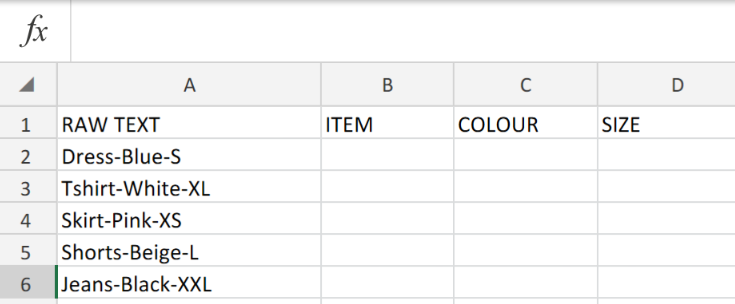Figure 2. of the Raw Text String.

1. Our objective here is to split the Text String in the first column into three separate categories in our Excel sheet.

To extract ITEM from our RAW TEXT String, we will use the Left Function. This will separate all characters that appear before the first hyphen on the left side of the RAW TEXT String.

### Generic Formula

`=LEFT(text,FIND(character,text)-1)`

• We will enter into cell B2 is as follows `=LEFT(A2,SEARCH("-",A2,1)-1)`
• This operation syntax should then be copied down the entire ITEM column.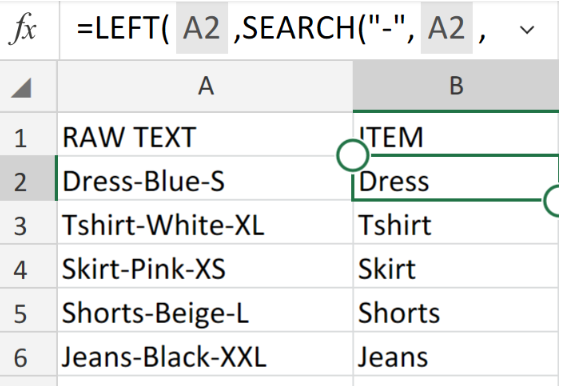Figure 3. of Left Function in Excel.

To extract COLOUR from our RAW TEXT String, will will use the Mid Function.

This will separate all characters that appear in the middle of the RAW TEXT String.

### Generic Formula

`=MID(text, FIND("-", text) + 1, FIND("-", text,FIND("-",text)+1) - FIND("-",text) - 1)`

• We will enter into cell C2 is as follows `=MID(A2,SEARCH("-",A2)+1,SEARCH("-",A2, SEARCH("-",A2)+1)-SEARCH("-",A2)-1)`
• This operation syntax should then be copied down the entire COLOUR column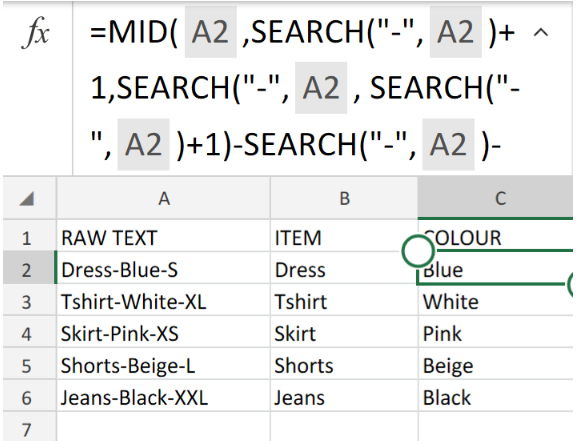Figure 4. of the Mid Function in Excel.

To extract SIZE from our RAW TEXT String, we will use the Right Function.

This will separate all characters that appear after the second hyphen of the RAW TEXT String.

### Generic Formula

`=RIGHT(text,LEN(text) - FIND("-", text, FIND("-", text) + 1))`

• We will enter into cell D2 is as follows `=RIGHT(A2,LEN(A2)-SEARCH("-",A2,SEARCH("-",A2)+1))`
• This operation syntax should then be copied down the entire SIZE column.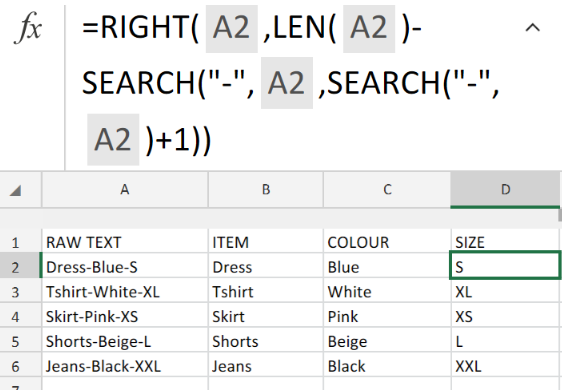Figure 5. of the Right Function in Excel.

In the formulas and examples given above, the frequently occurring numbers -1 and +1 are corresponding with the number of characters in the Raw Text String.

In our illustration, it is a hyphen. 1 character. If our Raw Text String consisted of 2 characters, e.g. a space and a coma, then we would use only the comma (“,”) for the SEARCH function, and then use -2 and +2 rather than -1 and +1.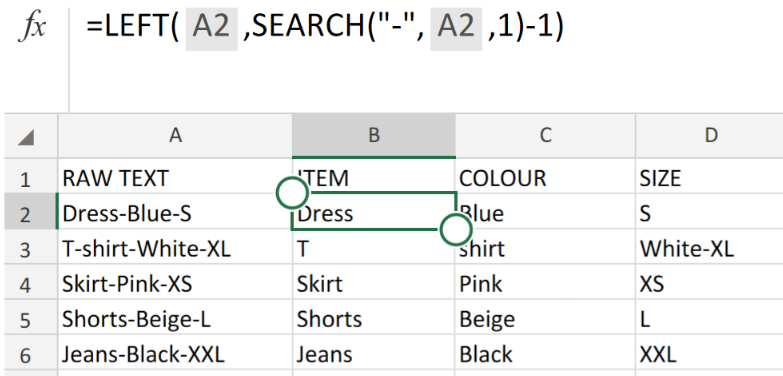Figure 6. of Final Result.

## Instant Connection to an Expert through our Excelchat Service

Our live Excelchat Service is here for you. We have Excel Experts available 24/7 to answer any Excel questions you may have. Guaranteed connection within 30 seconds and a customized solution for you within 20 minutes.

### Did this post not answer your question? Get a solution from connecting with the expert.Another blog reader asked this question today on Excelchat:## Subscribe to Excelchat.coAnother blog reader asked this question today on Excelchat: